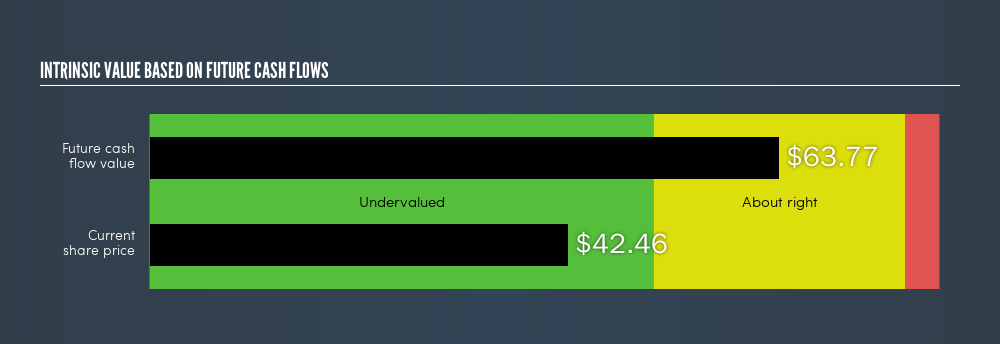latest

NRG Energy, Inc. (NYSE:NRG) Is Trading At A 33.42% Discount

Want to participate in a short research study? Help shape the future of investing tools and receive a \$20 prize!

I am going to run you through how I calculated the intrinsic value of NRG Energy, Inc. (NYSE:NRG) by estimating the company’s future cash flows and discounting them to their present value. I will use the discounted cash flows (DCF) model. Don’t get put off by the jargon, the math behind it is actually quite straightforward. If you want to learn more about discounted cash flow, the basis for my calcs can be read in detail in the Simply Wall St analysis model. If you are reading this and its not February 2019 then I highly recommend you check out the latest calculation for NRG Energy by following the link below.

Step by step through the calculation

I’m using the 2-stage growth model, which simply means we take in account two stages of company’s growth. In the initial period the company may have a higher growth rate and the second stage is usually assumed to have perpetual stable growth rate. In the first stage we need to estimate the cash flows to the business over the next five years. For this I used the consensus of the analysts covering the stock, as you can see below. The sum of these cash flows is then discounted to today’s value.

5-year cash flow forecast

 2019 2020 2021 2022 2023 Levered FCF (\$, Millions) \$2.17k \$1.33k \$1.24k \$1.16k \$1.09k Source Analyst x2 Analyst x3 Analyst x1 Est @ -5.96% Est @ -5.96% Present Value Discounted @ 8.55% \$2.00k \$1.13k \$968.01 \$838.61 \$726.51

Present Value of 5-year Cash Flow (PVCF)= US\$5.7b

After calculating the present value of future cash flows in the intial 5-year period we need to calculate the Terminal Value, which accounts for all the future cash flows beyond the first stage. For a number of reasons a very conservative growth rate is used that cannot exceed that of the GDP. In this case I have used the 10-year government bond rate (2.7%). In the same way as with the 5-year ‘growth’ period, we discount this to today’s value at a cost of equity of 8.5%.

Terminal Value (TV) = FCF2023 × (1 + g) ÷ (r – g) = US\$1.1b × (1 + 2.7%) ÷ (8.5% – 2.7%) = US\$19b

Present Value of Terminal Value (PVTV) = TV / (1 + r)5 = US\$19b ÷ ( 1 + 8.5%)5 = US\$13b

The total value is the sum of cash flows for the next five years and the discounted terminal value, which results in the Total Equity Value, which in this case is US\$18b. To get the intrinsic value per share, we divide this by the total number of shares outstanding, or the equivalent number if this is a depositary receipt or ADR. This results in an intrinsic value of \$63.77. Relative to the current share price of \$42.46, the stock is quite undervalued at a 33% discount to what it is available for right now.NYSE:NRG Intrinsic value, February 22nd 2019

Important assumptions

The calculation above is very dependent on two assumptions. The first is the discount rate and the other is the cash flows. You don’t have to agree with my inputs, I recommend redoing the calculations yourself and playing with them. Because we are looking at NRG Energy as potential shareholders, the cost of equity is used as the discount rate, rather than the cost of capital (or weighed average cost of capital, WACC) which accounts for debt. In this calculation I’ve used 8.5%, which is based on a levered beta of 0.800. This is derived from the Bottom-Up Beta method based on comparable companies, with an imposed limit between 0.8 and 2.0, which is a reasonable range for a stable business.

Next Steps:

Although the valuation of a company is important, it shouldn’t be the only metric you look at when researching a company. What is the reason for the share price to differ from the intrinsic value? For NRG, there are three relevant aspects you should further research:

1. Financial Health: Does NRG have a healthy balance sheet? Take a look at our free balance sheet analysis with six simple checks on key factors like leverage and risk.
2. Future Earnings: How does NRG’s growth rate compare to its peers and the wider market? Dig deeper into the analyst consensus number for the upcoming years by interacting with our free analyst growth expectation chart.
3. Other High Quality Alternatives: Are there other high quality stocks you could be holding instead of NRG? Explore our interactive list of high quality stocks to get an idea of what else is out there you may be missing!

PS. The Simply Wall St app conducts a discounted cash flow for every stock on the NYSE every 6 hours. If you want to find the calculation for other stocks just search here.

We aim to bring you long-term focused research analysis driven by fundamental data. Note that our analysis may not factor in the latest price-sensitive company announcements or qualitative material.

If you spot an error that warrants correction, please contact the editor at editorial-team@simplywallst.com. This article by Simply Wall St is general in nature. It does not constitute a recommendation to buy or sell any stock, and does not take account of your objectives, or your financial situation. Simply Wall St has no position in the stocks mentioned. Thank you for reading.